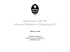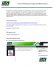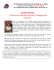axis major reduced regression rma

Transcription

axis major reduced regression rma
Scaling in Biology
Lecture 2
1/24/07
-Statistical Tool Kit
-Regression models
-Calculating regression parameters
- Critical Assumptions
-Importance of error
-Alternative regression models
What is scaling?
How attributes of a system change with changes in dimension
- a functional relationship!
A change in the basic physical quantities
(Mass, Length, Time, Temp)
MLT (T)
•Changes in organismal mass, length, volume
•Changes in spatial extent (area)
•Changes in time (temporal dynamics)
•Variation in temperature
Physical measurements: Energy, density, power, velocity etc.
can be broken down to units of MLT
Dimensional Analysis
Scaling Analysis - Building a tool kit
“If I vary X how does Y change?”
We are interested in functional relationship between X and Y
How do we assess the functional relationship?
Many ways
Simplest model - linear or power function
A central tool in scaling studies is the regression model
One Example:
How does a change in organismal size influences trait Y?
-Measure mass or length (X), measure response variable (Y)
-Characterize functional relationship - regression model
Plot data . . . . .
-relationship non-linear?
-transform data . . . .
Log transform data (Log10) - relationship a straight line?
Why log transform?
Statistical justification . . . .
Standard parametric statistics assume residual variation is
normally distributed.
Transform allometric data residuals then tend to be normally
distributed.
- can use parametric statistics based on gaussian
distributions.
Fit regression model to characterize functional relationship
Deeper issues:
Reveals Fundamental Aspects of Biology . . .
Some may say that log-log plots “hide important variation”
Not a correct statement . . . .
On log transformed axes - a constancy of residual variation
means that for a given value of X the proportion of variation
not explained by Y is proportionally constant (!)
This is an important insight!
Only by log transformation can one clearly document this
Remember:
Power-relationships and constant proportions of variance
point to importance of multiplicative (not additive)
processes in biology.
Important - many scaling attributes are non-linear!
A log scale allows one to view the multiplicative
nature of biological phenomena
Biology is often multiplicative
and rarely (?) additive . . .
A 1g change in the mass of a mouse is large but it is miniscule for an elephant!
Regression Tutorial
Diameter(cm) Tracheid diam
5
1.2
2
3
7.5
10.2
30
48
57
17
13
9
4.5
2.8
4
3.8
4
5
7
7.3
14
15
16.7
34.8
22
14
17
19
27
30
38
44
45
32
29
26.8
21
16
18
19
20
23
25
28
30
29.5
30
36.8
Allometric study of variation in
plant xylem tracheid dimensions
Traceid Diam. (um)
50
40
30
20
Stem Diam (cm)
Xi
Yi
Diameter(cm) Tracheid diam
5
1.2
2
3
7.5
10.2
30
48
57
17
13
9
4.5
2.8
4
3.8
4
5
7
7.3
14
15
16.7
34.8
22
14
17
19
27
30
38
44
45
32
29
26.8
21
16
18
19
20
23
25
28
30
29.5
30
36.8
Log10 Diameter Log10 Tracheid Diam
0.69897
0.07918125
0.30103
0.47712125
0.87506126
1.00860017
1.47712125
1.68124124
1.75587486
1.23044892
1.11394335
0.95424251
0.65321251
0.44715803
0.60205999
0.5797836
0.60205999
0.69897
0.84509804
0.86332286
1.14612804
1.17609126
1.22271647
1.54157924
1.34242268
1.14612804
1.23044892
1.2787536
1.43136376
1.47712125
1.5797836
1.64345268
1.65321251
1.50514998
1.462398
1.42813479
1.32221929
1.20411998
1.25527251
1.2787536
1.30103
1.36172784
1.39794001
1.44715803
1.47712125
1.46982202
1.47712125
1.56584782
60
50
40
30
20
10
0
10
Log-Log plot
1.7
50
1.6
40
1.5
1.4
30
1.3
20
1.2
Stem Diam (cm)
60
50
40
30
20
10
0
10
1.1
0
0.5
1
1.5
Log 10 Stem Diameter
Statistical Fit of a Regression Model
There are several types of linear regression models
Model I (Least Squares)
Model II (Major Axis, Reduced Major Axis)
OLS Bisector Technique
Principle components, independent contrasts . . .
Each differs in assumptions of where the distribution of
‘error’ resides between the two variables of interest.
Important issue for characterizing scaling slope and
constant
Any functional relationships in biology
2
Slope Model I (or Least Squares i.e. LS) regression
Most regression packages are Model I
(ordinary least squares) regression
OLS - historically has been used
- Fit best-fit line through data which passes through
mean values of Y and X
- OLS regression minimizes the sum of squares of the deviations
of observed Y values from the major axis to fit the major axis
through average of X and Y.
- In OLS regression the deviations of observed values are parallel
to the Y-axis of the bivariate plot.
Overview of OLS Regression
OLS regression - Y on X minimizes (Y ’)2 and (Y ’’)2
OLS regression - X on Y minimizes (X ’)2 and (X ’’)2
Beware of stats packages!!!
Good
SAS
S+/R
JMP
SPSS
Beware . . .
Microsoft Excel
Canned graphing
programs
Critical variables for calculating regression parameters
Sample Variance
Number of
X values
Standard deviation
s X2 =
n
1
(xi ! x )2
"
n ! 1 i =1
Average of
all x values
A given x value
1 n
s =
(yi ! y )2
"
n ! 1 i=1
2
Y
SX =
s 2X
n
Sample Covariance
"(x
i
SXY =
! x )( yi ! y )
i =1
n !1
Calculate ‘Pearson product moment correlation coeficient’
Sample covariance
SXY
Sx SY
r=
Std deviation of X and Y
If fit is 100% then r = 1 (no residual variation)
Fitted Linear regression model
slope
intercept
Yi = ! LS + " LS Xi
Yi = ! LS + "LS Xi + #
Empirical measurements have error
The LS line ‘chooses’ values of !LS and ß LS that
minimizes
n
\$ {Y ! ("
i
i =1
LS + # LS Xi )}
2
Slope
! LS
Y " Y )( X " X )
(
S
#
=
=r
S
# (X " X )
i
i
i
2
i
Intercept
i
Y
X
!LS = Y " #LS X
Remember - intercept is log transformed!
Tracheid diameter (µm)
1.7
1.6
1.5
1.4
1.3
1.2
y =1.123 + 0.308x
r = 0.980
1.1
0
0.5
1
1.5
2
Log 10 Stem Diameter
Calculate the Confidence Intervals for slope and intercept
(95% CI when alpha = 0.05)
Calculate the standard error (SE)
SE =
Standard Deviation
n
SX =
Degrees of Freedom (df)
!
For a regression model df = n - 2
s 2X
Calculate Confidence intervals, OLS regression
[
CI = [P + t
]
SE ]
CI = P ! t" (df ) SEP
! (df )
(95% confidence limits t0.05(df))
P
P denotes parameter of interest (ßLS, !LS)
SE = Standard error of regression parameter
Test for ßLS = 0
or ßLS = predicted value of a priori model
http://shazam.econ.ubc.ca/intro/critval.htm
Will give you values for critical t
Assumptions of OLS Regression
Values of X do not randomly vary
(X is measured without error!)
The expected relationship between Y and X is linear
(Y = ! + " X).
Values of Y for any specified value of X are independently and
normally distributed Yi = " + ! Xi + #i
-where # is the random deviation of the error term
-which is assumed to be normally distributed with a
mean value equal to zero
(Residual deviation from fitted LS line normally distributed)
Samples of Y along the regression line have a common
variance (\$2 ) that is the variance of #i
(the variance is independent of the magnitude of Y or X)
Assumes each X value is unique (X varies independently).
For each value of Xi however, values of Yi are not fixed
by investigator -but- instead vary randomly such that they
have a normal distribution about Xi.
i.e. Xi is known exactly so that there is
no measurement error in Xi. All measurement
error is in Yi
There are times when OLS assumptions may not hold
May be error in measurements of Xi
What to do?
Alternative regression models
Model II regression (or principle axis regressions)
(Major Axis, Reduced Major Axis - focus on RMA)
-As in OLS regression the criteria for establishing the major axis)
through X and Y minimize the sum of squares.
-Fit the major axis through
Y and X
Model II regression (or principle axis regressions)
(Major Axis, Reduced Major Axis - focus on RMA)
-As in LS regression the criteria for establishing the major axis)
through X and Y minimize the sum of squares.
-Fit the major axis through
Y and X
In OLS regression the deviations of observed values are
parallel to the Y-axis of the bivariate plot (no error in Xi).
Model II regression - the deviations are perpendicular to the
regression line established by the major axis regression line
(Measurement error likely in values of Xi and Yi).
Summary of Regression Models
Two major types of Model II Regression
(SMA or standard axis regression)
Major axis regression
Minimizes the sum of
the distances (z’)2 and (z’’)2
(MA major axis regression)
Reduced major axis
regression
Minimizes the sum
of the products (x`y’) and (x’’y’’)
From Warton et al. 2006
(or OLS)
(or RMA)
(or Major axis regression)
Summary
Model I (OLS regression)
-Deviations of observed values of Y are parallel
to the Y -axis (all error on Y axis).
Model II (RMA regression etc.)
- Deviations are perpendicular to the
regression line
(error in both Y AND X).
Why important? Implications for assessing the functional
relationship between X and Y
Model II Regression (RMA)
Easy to calculate by hand . . . .
Slope
SX
! RMA =
SY
Standard deviation of X or Y
Intercept
! RMA = Y " # RMA X
Confidence intervals
Some differing thoughts on this but . . .
use same confidence intervals from OLS and adjust to new
value for slope and intercept (see Sokal and Rolf)
The numerical value of RMA will always be greater than
that of the LS scaling exponent because
ßRMA = ßOLS/rOLS
Remember to use r and not r2!
If the r2 value is high then the difference between OLS Model I and
Model II regression will be small to negligent.
"RMA = "LS/r
1.7
1.6
= 0.308/0.980
1.5
= 0.314
1.4
1.3
1.2
y =1.123 + 0.308x
"LS = 0.308
r = 0.980
1.1
0
0.5
1
1.5
Log 10 Stem Diameter
2
OLS Bisector Method
Isobe et al.
Relation between RMA and
First principle component . .
OLS bisector performs better than Model II
Which regression model to use??
Model I vs. Model II
- If error is suspected in X (average size, max size etc.) then
Model II is preferable.
- If range in X is large (more than 2 orders of magnitude) then
OLS regression seems to be fine. However, if less than
Two orders of magnitude then at least report both OLS and
RMA . . . . RMA is likely preferable.
Why? Measurement error in X may be
relatively larger than residual variation in Y
- If you have no a priori expectation what is the dependent
or independent variable then RMA preferable
-If the units in both X and Y are the same (i.e. mass vs. mass)
then RMA is likely best.
- NOTE! if value of r is high choice really does not matter
(Body size is usually measured with little error)
Which RMA model to use?
Stay tuned . . . Hot statisticians are on it.
For now . . . When in doubt RMA.
Class Regression Exercise in R/S+
http://eeb37.biosci.arizona.edu/~brian/teaching.html
Calculate regression models for two datasets
(1) Using plant xylem dataset calculate slope
and intercept for OLS and RMA by hand
Can you calculate the 95% CI ?
(2) Using U.S. record tree size dataset
and utilizing R/S+ calculate OLS,
RMA, Bisector regression models.
http://eeb37.biosci.arizona.edu/~brian/splus.html
http://web.maths.unsw.edu.au/~dwarton/programs.html
http://www.bio.sdsu.edu/pub/andy/rma.html
http://cran.r-project.org/src/contrib/Descriptions/smatr.html
Ricker, W.E. 1973. Linear regressions in fishery research. Journal of the
Fisheries Research Board of Canada. 30:409-434.
Warton D.I., Wright I.J., Falster D.S. & Westoby M. (2006) Bivariate line-fitting methods
for allometry. !Biological Reviews 81, 259-291.

Similar documents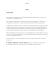Level 2 Computing — Project 2: Linear Regression Analysis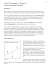The Multiple Linear Regression Model 2 Short Guides to Microeconometrics Kurt Schmidheiny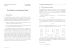Poster - University of Sussex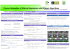GERTIFICATE M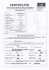Presentation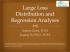texas calculatem key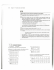rma request form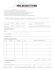Lecture 1 - Biostatistics